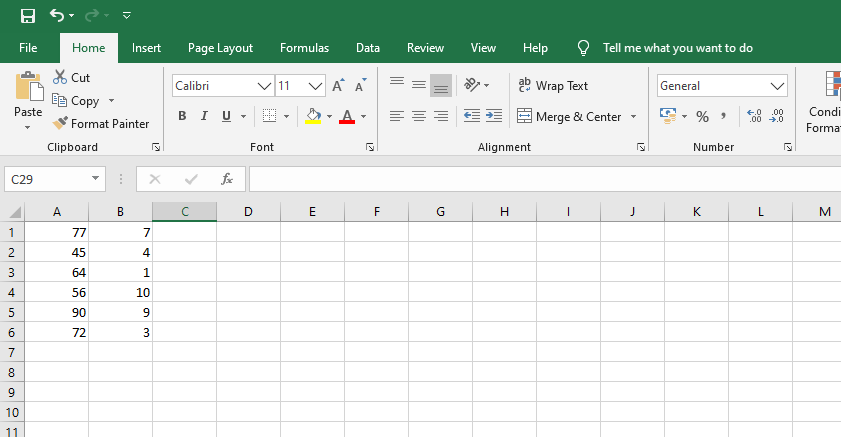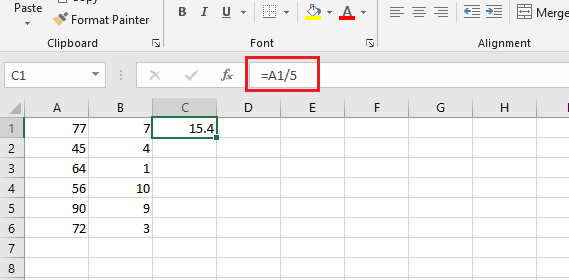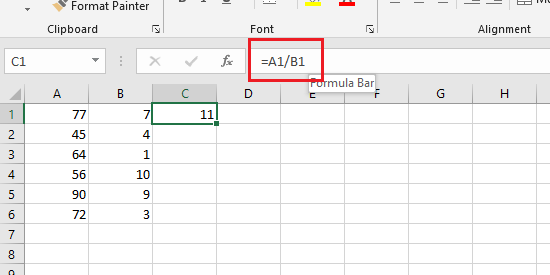# What Is The Formula For Division In Excel?

Microsoft Excel provides users with several different methods of dividing numbers and values. What you will not see the the division symbol (÷) we normally use to divide numbers in mathematics.

The official division symbol in Microsoft Excel is the forward slash (/). Different scenarios and data calls for different formula to divide in Excel. But the symbol that represents ‘divide’ is the forward slash symbol.

Like all other basic math operations, the formula for division in Excel starts with the equal sign (=) and is placed in the formula section at the top of the spreadsheet.

## Formula For Division In ExcelIn the simple example above,  you can have several different variation of the formula for division in Excel depending on what you want to achieve and just how you want to work. But everything revolves around the same (/) symbol used for division in Excel.

You can choose to have the values in column C be the values of column A divided by 3. In that case, your formula could be either consist of the values inside the cells or the cells themselves.

To initiate the formula for division in Excel, just select the cell where you want your final value to be, then press the equals (=) sign and the formula section in the spreadsheet will be activated. Then you can type in your formula.

Example 1:

For instance, in cell C1,  if I want the value to be the value of A1 divided by 5, I can easily add that in the formula. Just select the call C1 and tap on the equals (=) sign and complete the formula as follows.

= A1/5Pressing the Enter key on your keyboard let’s Excel do that calculation and place the answer in the cell you selected.

Example 2:

You can also have values in one cell divided by values in another cell. For instance, you might want the values in the C column to be the result of dividing the values in the a column by the values in the b column. For this scenario, here’s your formula for division in Excel.

Select the C1 cell with your mouse,  press ‘=‘ on your keyboard and type the following

=A1/B1

####To avoid errors when typing, Excel lets you pount the cells instead of typing the call numbers into the formula. All you need to do is select the cell where you want your answer, then press (=) on the keyboard.  When the formula bar is activated, click on cell A1 with your mouse, then press the division (/) symbol, and finally select cell B1 with your mouse. Then press ENTER on your keyboard to get the answer.

Also read: How to Convert Excel to PDF